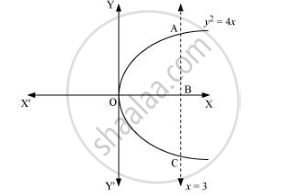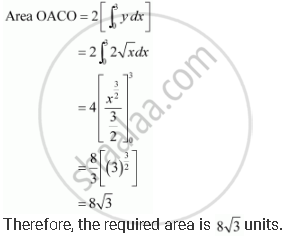Share

# Find the Area of the Region Bounded by the Curve Y2 = 4x And the Line X = 3 - CBSE (Commerce) Class 12 - Mathematics

#### Question

Find the area of the region bounded by the curve y2 = 4x and the line x = 3

#### Solution

The region bounded by the parabola, y2 = 4x, and the line, x = 3, is the area OACO.The area OACO is symmetrical about x-axis.

∴ Area of OACO = 2 (Area of OAB)Is there an error in this question or solution?

#### APPEARS IN

NCERT Solution for Mathematics Textbook for Class 12 (2018 to Current)
Chapter 8: Application of Integrals
Q: 11 | Page no. 366
Solution Find the Area of the Region Bounded by the Curve Y2 = 4x And the Line X = 3 Concept: Area Under Simple Curves.
S